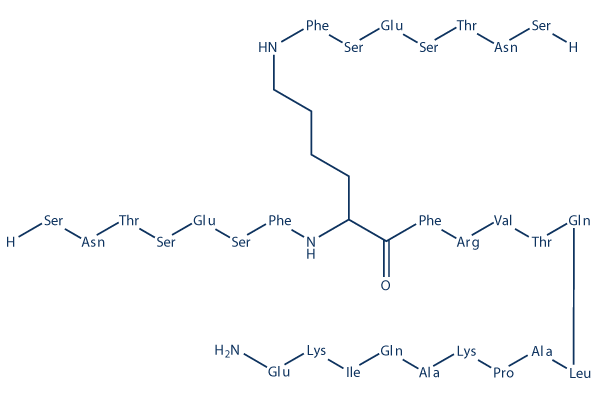# AUNP-12

For research use only.

Catalog No.S8549 Synonyms: Aur-012, Aurigene-012, Aurigene NP-12

1 publicationCAS No. 1353563-85-5

AUNP-12 (Aur-012, Aurigene-012, Aurigene NP-12), a new immune checkpoint modulator, is an inhibitor of the PD-1 pathway.

### Biological Activity

Description AUNP-12 (Aur-012, Aurigene-012, Aurigene NP-12), a new immune checkpoint modulator, is an inhibitor of the PD-1 pathway.
Targets
 PD-L1 (Cell-free assay)
In vitro

AUNP-12 displays an EC50 = 0.72 nM in the inhibition of binding PD1 to PD-L2 using hPDL2 expressing HEK293 cells, and an EC50 = 0.41 nM in a rat peripheral blood mononuclear cells (PBMC) proliferation assay using hPDL1 expressing MDA-MB231 cells.

### Solubility (25°C)

 In vitro Water 100 mg/mL (30.66 mM) Ethanol '-1 mg/mL

* Please note that Selleck tests the solubility of all compounds in-house, and the actual solubility may differ slightly from published values. This is normal and is due to slight batch-to-batch variations.

Molecular Weight 3261.55 C142H226N40O48 1353563-85-5 3 years-20°C powder 2 years-80°C in solvent Aur-012, Aurigene-012, Aurigene NP-12 CCC(C)C(C(=O)NC(CCC(=O)N)C(=O)NC(C)C(=O)NC(CCCCN)C(=O)N1CCCC1C(=O)NC(C)C(=O)C(=O)C(CC(C)C)NC(=O)C(CCC(=O)N)NC(=O)C(C(C)O)NC(=O)C(C(C)C)NC(=O)C(CCCNC(=N)N)NC(=O)C(CC2=CC=CC=C2)NC(=O)C(CCCCNNC(CC3=CC=CC=C3)C(=O)NC(CO)C(=O)NC(CCC(=O)O)C(=O)NC(CO)C(=O)NC(C(C)O)C(=O)NC(CC(=O)N)C(=O)NC(CO)C=O)NC(=O)C(CC4=CC=CC=C4)NC(=O)C(CO)NC(=O)C(CCC(=O)O)NC(=O)C(CO)NC(=O)C(C(C)O)NC(=O)C(CC(=O)N)NC(=O)C(CO)N)NC(=O)C(CCCCN)NC(=O)C(CCC(=O)O)NN

### In vivo Formulation Calculator (Clear solution)

 Step 1: Enter information below (Recommended: An additional animal making an allowance for loss during the experiment) Dosage mg/kgAverage weight of animals gDosing volume per animal ulNumber of animals Step 2: Enter the in vivo formulation (This is only the calculator, not formulation. Please contact us first if there is no in vivo formulation at the solubility Section.) % DMSO+ % PEG300 Corn oil + % Tween 80+ % ddH2O CalculateReset

### Bio Calculators

Molarity Calculator

## Molarity Calculator

Calculate the mass, volume or concentration required for a solution. The Selleck molarity calculator is based on the following equation:

### Mass (mg) = Concentration (mM) × Volume (mL) × Molecular Weight (g/mol)

• Mass
Concentration
Volume
Molecular Weight

*When preparing stock solutions, please always use the batch-specific molecular weight of the product found on the via label and SDS / COA (available on product pages).

Dilution Calculator

## Dilution Calculator

Calculate the dilution required to prepare a stock solution. The Selleck dilution calculator is based on the following equation:

### Concentration (start) x Volume (start) = Concentration (final) x Volume (final)

This equation is commonly abbreviated as: C1V1 = C2V2 ( Input Output )

• C1
V1
C2
V2

* When preparing stock solutions always use the batch-specific molecular weight of the product found on the vial label and SDS / COA (available online).

## The Serial Dilution Calculator Equation

• ### Computed Result

• C1=C0/X C2=C1/X C1: LOG(C1): C2: LOG(C2): C3: LOG(C3): C4: LOG(C4): C5: LOG(C5): C6: LOG(C6): C7: LOG(C7): C8: LOG(C8):
Molecular Weight Calculator

## Molecular Weight Calculator

Enter the chemical formula of a compound to calculate its molar mass and elemental composition:

### Total Molecular Weight: g/mol

Tip: Chemical formula is case sensitive. C10H16N2O2 c10h16n2o2

#### Instructions to calculate molar mass (molecular weight) of a chemical compound:

To calculate molar mass of a chemical compound, please enter its chemical formula and click 'Calculate'.

#### Definitions of molecular mass, molecular weight, molar mass and molar weight:

Molecular mass (molecular weight) is the mass of one molecule of a substance and is expressed in the unified atomic mass units (u). (1 u is equal to 1/12 the mass of one atom of carbon-12)
Molar mass (molar weight) is the mass of one mole of a substance and is expressed in g/mol.

#### Molarity Calculator

 Mass Concentration Volume Molecular Weight pg ng μg mg g kg = fM pM nM μM mM M × nL μL mL L ×

### Tech Support

Answers to questions you may have can be found in the inhibitor handling instructions. Topics include how to prepare stock solutions, how to store inhibitors, and issues that need special attention for cell-based assays and animal experiments.

Tel: +1-832-582-8158 Ext:3